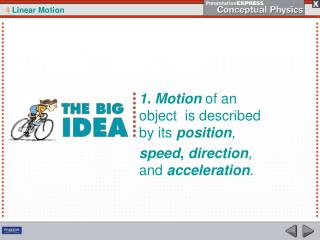Download Presentation1. Motion of an object is described by its position ,

Loading in 2 Seconds...

# 1. Motion of an object is described by its position , - PowerPoint PPT Presentation

1. Motion of an object is described by its position , speed , direction , and acceleration. 2) MKS units are M eters, K ilograms S econds. 3. Initial means “ first ” or “ starting ”. 4 . D = “ delta ” D means “ the change in ” 5. D = final – initial.I am the owner, or an agent authorized to act on behalf of the owner, of the copyrighted work described.
Download Presentation## 1. Motion of an object is described by its position ,

An Image/Link below is provided (as is) to download presentation

Download Policy: Content on the Website is provided to you AS IS for your information and personal use and may not be sold / licensed / shared on other websites without getting consent from its author.While downloading, if for some reason you are not able to download a presentation, the publisher may have deleted the file from their server.

- - - - - - - - - - - - - - - - - - - - - - - - - - E N D - - - - - - - - - - - - - - - - - - - - - - - - - -
Presentation Transcript
1. 1. Motion of an object is described by its position, speed, direction, and acceleration.

2. 2) MKS units areMeters, Kilograms Seconds

3. 3. Initial means “first” or “starting”

4. 6. wrt means “with respect to”

5. 7. fixed means constant, unchanging.

6. When is an object moving?

7. 4.1Motion Is Relative 8. An object is moving when its position changes relative to a fixed point.

8. Vectors and Scalars

9. 4.1Motion Is Relative 9 . Vectors or vector quantities have magnitude and direction • Scalars or scalar quantities have only magnitude

10. 11. Magnitude means size

11. 4.1Motion Is Relative 12.Distance means total path length an object travels 13.Displacement means final position minus initial position

12. 4.1Motion Is Relative 14. In symbols Displacement =Dxor Dy

13. Travel around perimeter:

14. Travel around perimeter: from A B

15. Travel around perimeter: from A BC

16. Travel around perimeter: from A BC D

17. Travel around perimeter: from A BCDA

18. Distance = path length =

19. Distance = path length =28 m

20. Distance = path length =28 m Displacment = 0

21. 15) Distance is a scalar and Displacement is a vector

22. 16. Motion is relative: we always describe motion relative to something else

23. 4.1Motion Is Relative How can you tell if an object is moving?

24. http://www.youtube.com/watch?v=oRBchZLkQR0http://www.youtube.com/watch?v=KLd-rJMoekohttp://www.youtube.com/watch?v=xMF2CfYLomYhttp://www.youtube.com/watch?v=oRBchZLkQR0http://www.youtube.com/watch?v=KLd-rJMoekohttp://www.youtube.com/watch?v=xMF2CfYLomY

25. 4.2Speed 18. Speed = distance time v = d t

26. 4.2Speed 19. Before Galileo, people described motion as “slow” or “fast.”

27. 4.2Speed 20.Galileo was the first to calculate speed 21. Speed is how fast an object moves

28. 4.2Speed 22. MKS units of speed meters/second = m/sec . v = d t

29. 4.2Speed

30. 4.2Speed Instantaneous Speed 23. Instantaneous speed is the speed at an instant of time 24. Speedometer measures instantaneous speed.

31. 4.2Speed The speedometer gives readings of instantaneous speed in both mi/h and km/h.

32. 4.2Speed Average Speed 25. average speed = total distance total time.

33. 4.2Speed Is average speed the same as instantaneous speed?

34. REARRANGE equation: v = d/t 26. HOW FAR: total distance d = average speed X total time d = v • t

35. 27. Odometer measures how far an object travels.

36. 4.2Speed How can you calculate speed?

37. 4.3Velocity 28. Velocity is speed in a specific direction.

38. 4.3Velocity 29. MKS units of Velocity : m/sec

39. 4.3Velocity 30. Velocity is the rate of change of position V = Dx Dt

40. 4.3Velocity 31. Rate always means wrttime

41. 4.3Velocity 32. Speed is a scalar; and Velocity is a vector.

42. 4.3Velocity Constant Velocity 33. Constant velocity means traveling in a straight line at constant speed.

43. 4.3Velocity Changing Velocity 34. Velocity changes if speed changes or direction changes or both change .

44. 4.3Velocity The car on the circular track may have a constant speed but not a constant velocity, because its direction of motion is changing every instant.

45. 4.3Velocity How is velocity different from speed?

46. 35. Acceleration = change in velocitytime intervala = DVDT

47. 36. Acceleration is the rate of change of velocity.

48. 4.4Acceleration 37. Acceleration means decreases or increases in velocity. 38. Decreased acceleration is called deceleration.